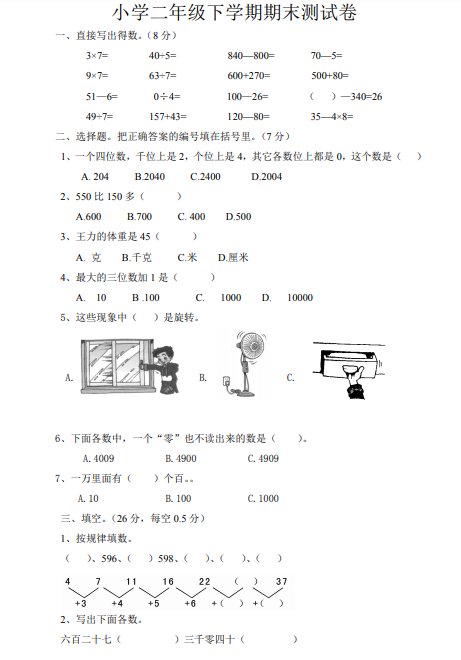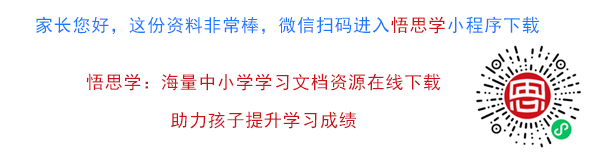3×7= 40÷5= 840—800= 70—5=
9×7= 63÷7= 600+270= 500+80=
51—6= 0÷4= 100—26= （ ）—340=26
49÷7= 157+43= 120—80= 35—4×8=

1、一个四位数，千位上是 2，个位上是 4，其它各数位上都是 0，这个数是（ ）
A. 204 B.2040 C.2400 D.2004

【PDF文档4页】最新版人教版小学二年级下册数学期末真题A4电子版资料_可直接打印_会员免费下载##### 评论信息